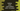# Python program to print a box pattern with plus at the center## Python program to print a box pattern with plus at the center:

In this post, we will learn how to print a box pattern with a plus or + at the center using Python. This program will take the row and column values as inputs from the user.

You can use numbers or any other character to print these patterns. We will learn how to use numbers and how to use characters like star or any other character to print it.

### Patterns for even and odd values:

This pattern will look different for even and odd lengths. For example, suppose we are using 0 to print the plus and 1 to print the box.

For row and column size 5, it will print the below pattern:

``````11011
11011
00000
11011
11011``````

For row and column size 4, it will print:

``````1001
0000
0000
1001``````

For even values, it will print two lines in the middle and for odd values, it will print one line. It is because for odd numbers, we can have a mid-point. But, for even numbers, we need two numbers as the mid.

## Algorithm:

We will use the below algorithm in the program:

• Take the row and column values as input from the user.
• Find the half of these values.
• Run two for loops. The outer loop will point to each row of the box and the inner loop will print the values for the box.
• We are using 0 to print the plus and 1 to print the box.
• If the outer loop is pointing to the middle row, print 0 or if the inner loop is pointing to the middle column, print 0.
• If the row is an even value, print 0 one row before the middle row. For example, if the row count is 6, the middle value will be 3. So, print 0 for both row number 3 and 2. Row and column numbers are starting from 0.
• For other values, print 1.

### Python program:

Below is the complete python program:

``````total_rows = int(input('Enter the number of rows: '))
total_cols = int(input('Enter the number of columns: '))

mid_point_row = int(total_rows / 2)
mid_point_col = int(total_cols / 2)

for i in range(0, total_rows):
for j in range(0, total_cols):
if i == mid_point_row or j == mid_point_col:
print('0', end='')
elif (total_rows % 2 == 0 and i == mid_point_row - 1) or (total_cols % 2 == 0 and j == mid_point_col - 1):
print('0', end='')
else:
print('1', end='')
print('')``````

Here,

• We are taking the total rows and total columns as inputs from the user and storing them in total_rows and total_cols variables.
• minpointrow and midpointcol are variables to store the mid points for both row and column.
• We are running two loops. The outer loop runs for total_rows number of times and the inner loop runs for total_cols number of times.
• Inside the inner loop, the if-elif-else statement is used to check and print the characters.
• It is printing 0 and 1 as we have discussed before in the algorithm.

### Output:

If you run this program, it will print output as like below:

``````Enter the number of rows: 6
Enter the number of columns: 6
110011
110011
000000
000000
110011
110011

Enter the number of rows: 5
Enter the number of columns: 5
11011
11011
00000
11011
11011``````

Instead of using numbers, you can also use any other character to print the pattern. For example,

``````total_rows = int(input('Enter the number of rows: '))
total_cols = int(input('Enter the number of columns: '))

mid_point_row = int(total_rows / 2)
mid_point_col = int(total_cols / 2)

for i in range(0, total_rows):
for j in range(0, total_cols):
if i == mid_point_row or j == mid_point_col:
print('*', end='')
elif (total_rows % 2 == 0 and i == mid_point_row - 1) or (total_cols % 2 == 0 and j == mid_point_col - 1):
print('*', end='')
else:
print('-', end='')
print('')``````

We can use this program to print patterns like this:

``````Enter the number of rows: 5
Enter the number of columns: 5
--*--
--*--
*****
--*--
--*--

Enter the number of rows: 15
Enter the number of columns: 15
-------*-------
-------*-------
-------*-------
-------*-------
-------*-------
-------*-------
-------*-------
***************
-------*-------
-------*-------
-------*-------
-------*-------
-------*-------
-------*-------
-------*-------``````